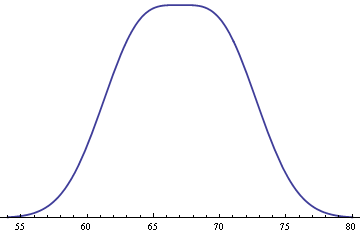# How flat is a normal mixture on top?

0
22Male and female heights both have a standard deviation of about 3 inches, with means of 70 inches and 64 inches. That’s a good first-pass model using round numbers.

If you ask what the height of an average adult is, not specifying male or female, you get a mixture of two normal distributions. If we assume an equal probability of selecting a male or female, then the probability density function for the mixture is the average of the density functions for men and women separately.

This mixture distribution is remarkably flat on top.

This happens whenever you have an equal mixture of two normal distributions, both having the same standard deviation σ, and with means 2σ apart. If the means were any closer together, the distribution would be rounder on top. If the means were any further apart, there would be a dip in the middle.

This makes sense intuitively if you think about what happens if you make things more extreme. If the means were as close together as possible, ie if they were the same, we’d simply have a normal distribution with its familiar curve on top. If the means were much further apart, we’d have two bumps that hardly appear to overlap.

See more on the application to heights here.

## Mathematical details

How flat is the mixture density on top? We can quantify the flatness by looking at a power series expansion centered at the peak.

To simplify matters, we can assume that σ = 1 and that the means of the two distributions are -μ and μ, with the primary interest being the case μ = 1. The probability density function for the mixture isIf we expand this into a Taylor series around 0 we getThis is a little complicated, but it explains a lot. Notice that the coefficient of x² has a term (μ² – 1).

We said above that if the means were any closer together than two standard deviations, the distribution would be rounder on top. That’s because if μ <1 then (μ² - 1) is negative and the curve is convex on top.

We also said that if the means were any further apart, there would be a dip in the middle. That’s because if μ> 1 then (μ² – 1) is positive and the curve is concave on top.

Now if μ = 1 then the x² term disappears. And because our function is even, its Taylor series only has terms with even powers. So if the x² term goes away the next term is not x3 but x4.

So one way to say how flat our curve is on top is that it is flat to within O(x4).

Let’s get more specific by evaluating the coefficients numerically. Too small xour mixture density function is

0.0965 – 0.0080 x4 + O(x6).

Now if x is small, x4 is extremely small, and the coefficient of 0.008 makes it even smaller.

.

Source